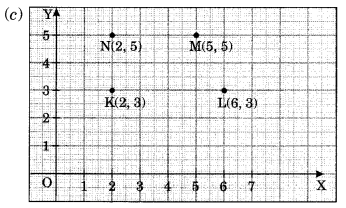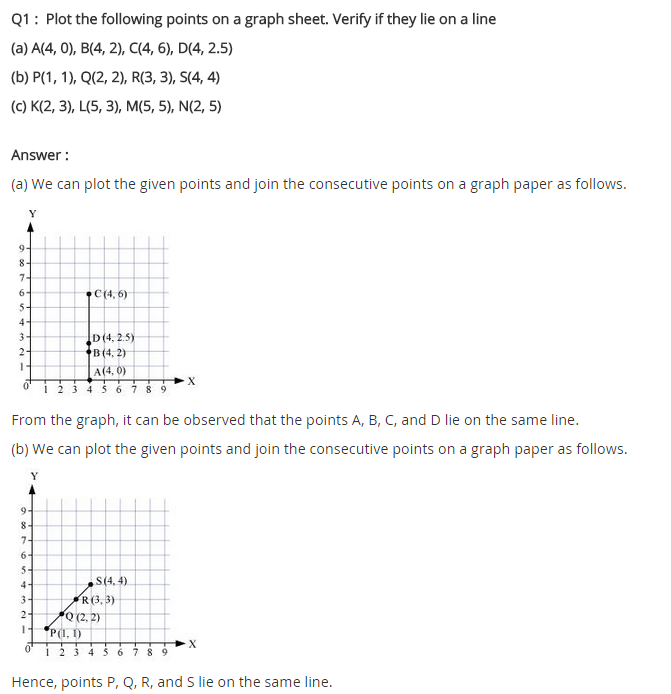# NCERT Solutions for Class 8 Maths Chapter 15 Introduction to Graphs Ex 15.2

NCERT Solutions for Class 8 Maths Chapter 15 Introduction to Graphs Ex 15.2

### NCERT Solutions for Class 8 Maths Chapter 15 Introduction to Graphs Exercise 15.2

Ex 15.2 Class 8 Maths Question 1.
Plot the following points on a graph sheet. Verify if they lie on a line.
(a) A (4, 0), B(4, 2), C(4, 6), D(4, 2.5)
(b) P(1, 1), Q(2, 2), R(3, 3), S(4, 4)
(c) K(2, 3), L(6, 3), M(5, 5), N (2, 5)
Solution:Yes, all the coordinate points lie on a line.Yes, all the coordinate points lie on a line.No, the coordinate points do not lie on a line.

Ex 15.2 Class 8 Maths Question 2.
Draw the line passing through (2, 3) and (3, 2). Find the coordinates of the points at which this line meets the x-axis and y-axis.Solution:
CD is the required line passing through the points A(2, 3), B (3, 2) which meets x-axis at C(5, 0) and y-axis at D(0, 5).

Ex 15.2 Class 8 Maths Question 3.
Write the coordinates of the vertices of each of these adjoining figures.Solution:
Required coordinates are as follows:
Coordinates of rectangle OABC are:
O(0, 0), A(2, 0), B(2, 3), C(0, 3)
Coordinates of parallelogram PQRS are:
P(4, 3), Q(6, 1), R(6, 5), S(4, 7)
Coordinates of triangle KLM are:
K(10, 5), L(7, 7), M (10, 8).

Ex 15.2 Class 8 Maths Question 4.
State whether True or False. Correct that is false.
(i) A point whose x-coordinate is zero and y-coordinate is non-zero will lie on the y-axis.
(ii) A point whose y-coordinate is zero and x-coordinate is 5 will lie on y-axis.
(iii) The coordinates of the origin are (0, 0).
Solution:
(i) True
(ii) False, the point will lie on the x-axis with coordinates (5, 0).
(iii) True## SabDekho

The Complete Educational Website